## 8.201 Rooted trees

• Rooted Trees

• A rooted tree is a directed tree with a distinguished vertex \$r4, called a root. Such that, for every vertex v there is a directed path from r to v.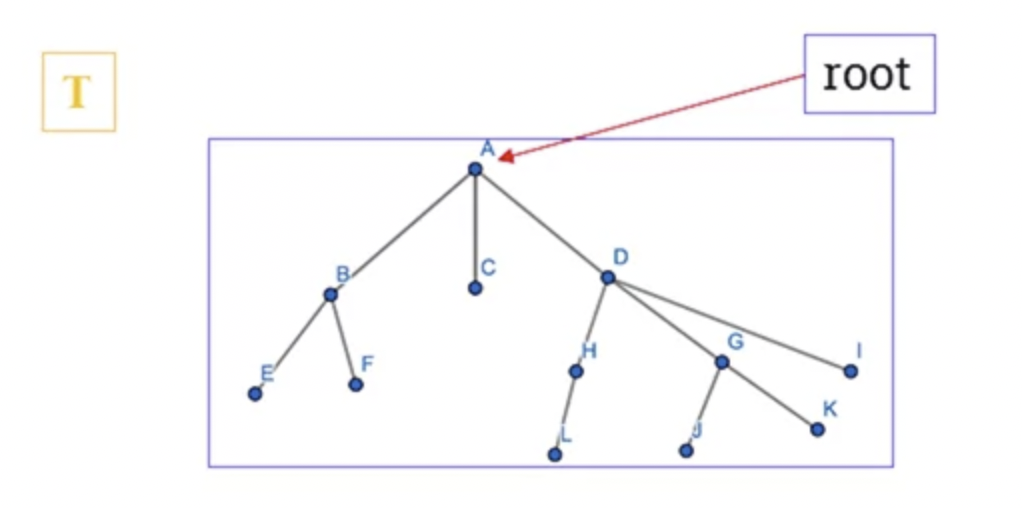• A directed tree is represented as a rooted tree if and only if one vertex has in-degree 0 whereas all other vertices have in-degree 1.

•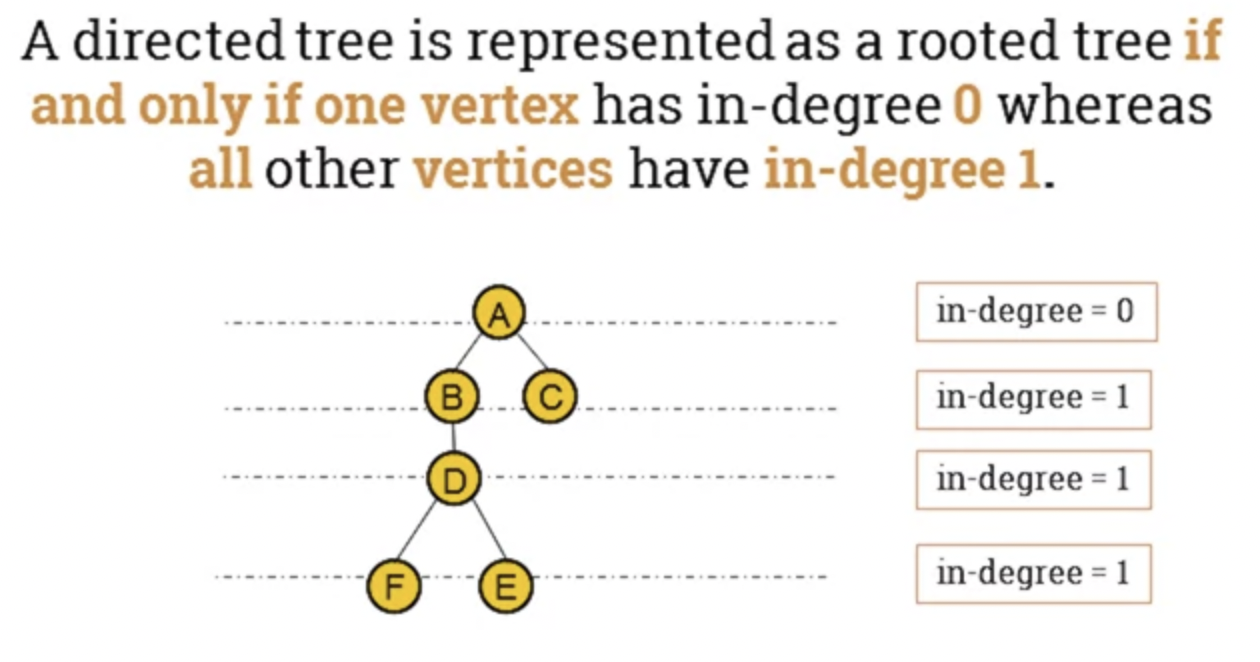• Terminology of rooted trees.
• In the image above:
• A is the root of the tree.
• B is called the parent of D.
• E and F are the children of D.
• B and A are ancestors of E and F (E and F are siblings)
• B and D are called internal nodes.
• C, E and F are called external nodes.
• Depth and height in a tree
• Depth or path length of a node in a tree is the number of edges from root to that node.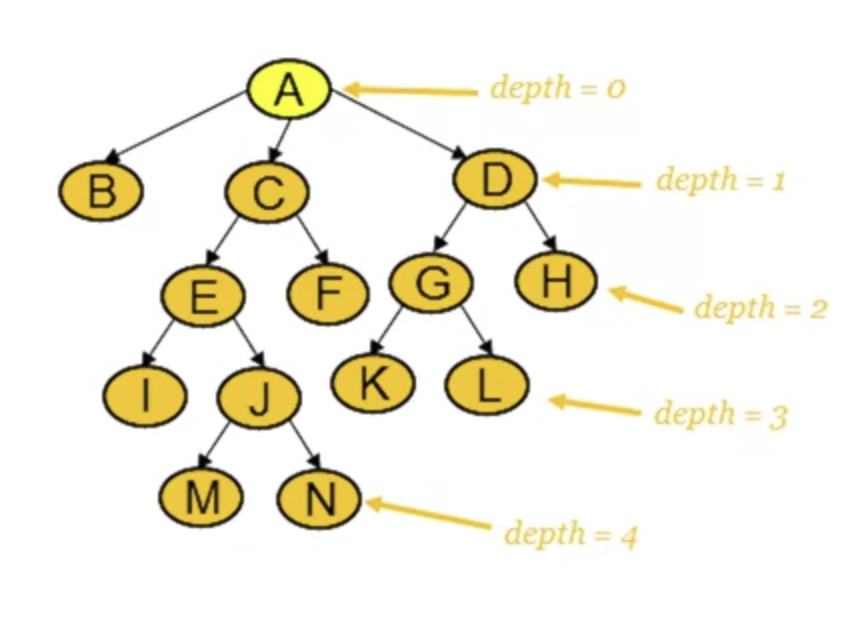• Height of a node in a tree is the longest path from that node to the leaf.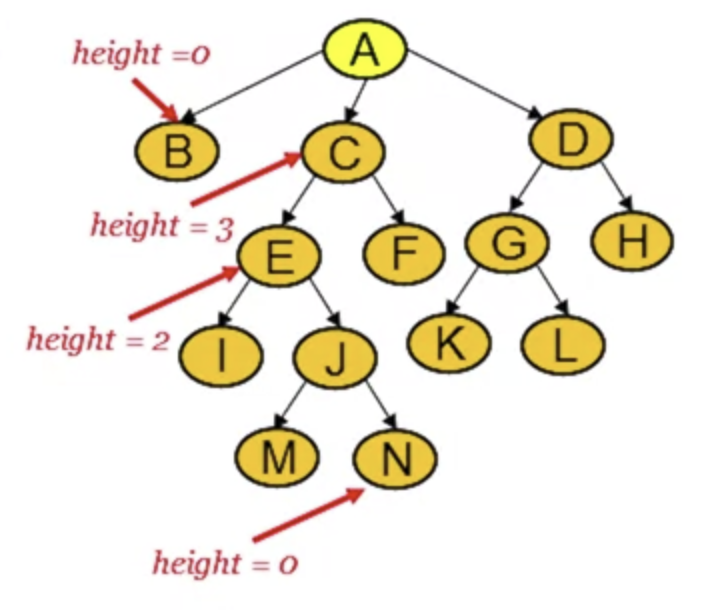• The depth or the height of a tree is the maximum path length across all nodes.
• The depth (height) of this tree is 4.
• Special trees
• Binary tree is a rooted tree in which every vertex has 2 or fewer children.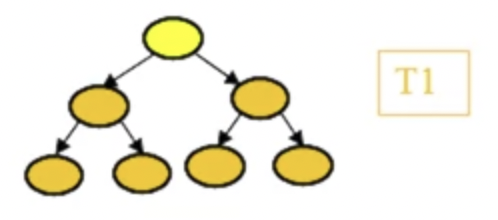• Ternary tree is a rooted tree in which every vertex has 3 or fewer children.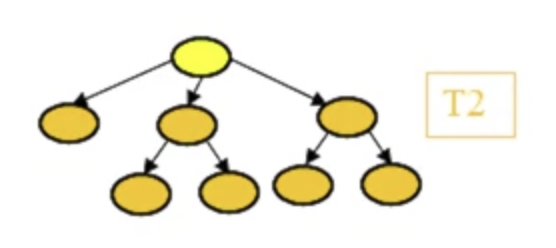• m-ary tree is a rooted tree in which every vertex has m or fewer children.
• Regular rooted trees
• A regular m-ary tree is regular if everyone of its node has exactly m children.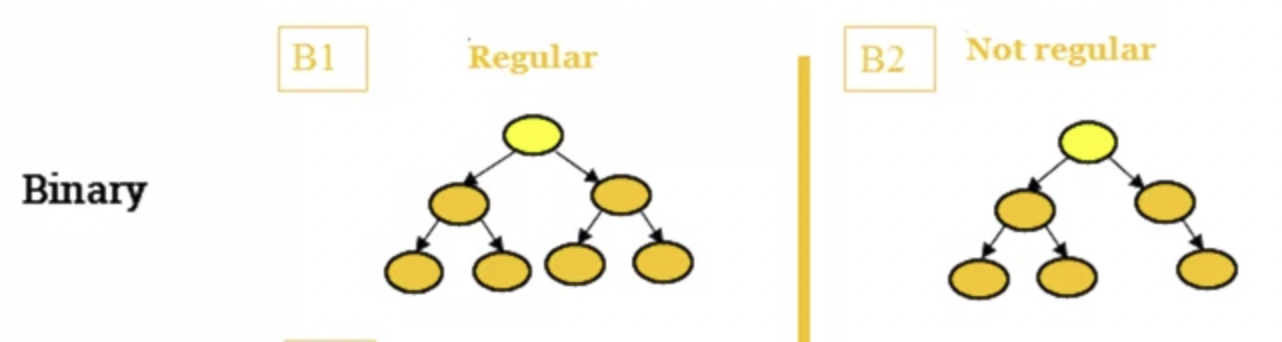• Properties
• m-ary tree has at most $m^h$ vertices as level h.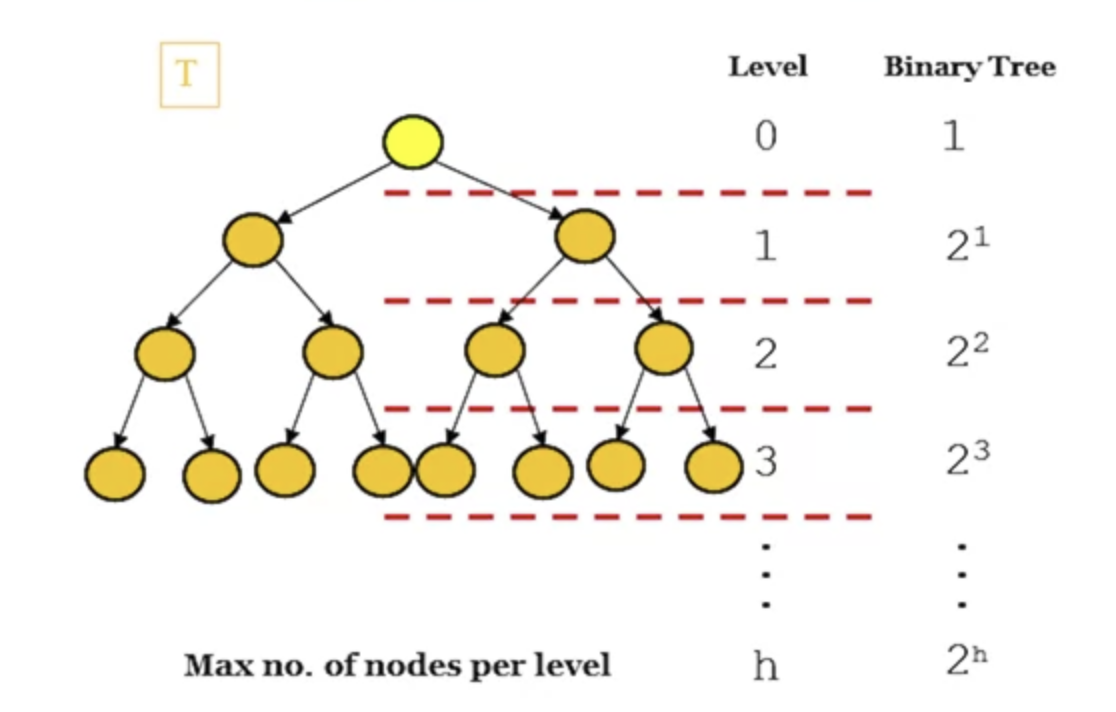• Isomorphic Trees

• 2 trees $T_1$ and $T_2$ are isomorphic if there is a bijection: $f: V(T_1) \rightarrow V(T_2)$ which preserves adjacency and non-adjacency.
• That is, if uv is an edge in $E(T_1)$ and $f(u)f(v)$ is in $E(T_2)$
• Notation
• $T_1 \cong T_2$ means that $T_1$ and $T_2$ are isomorphic.
• Example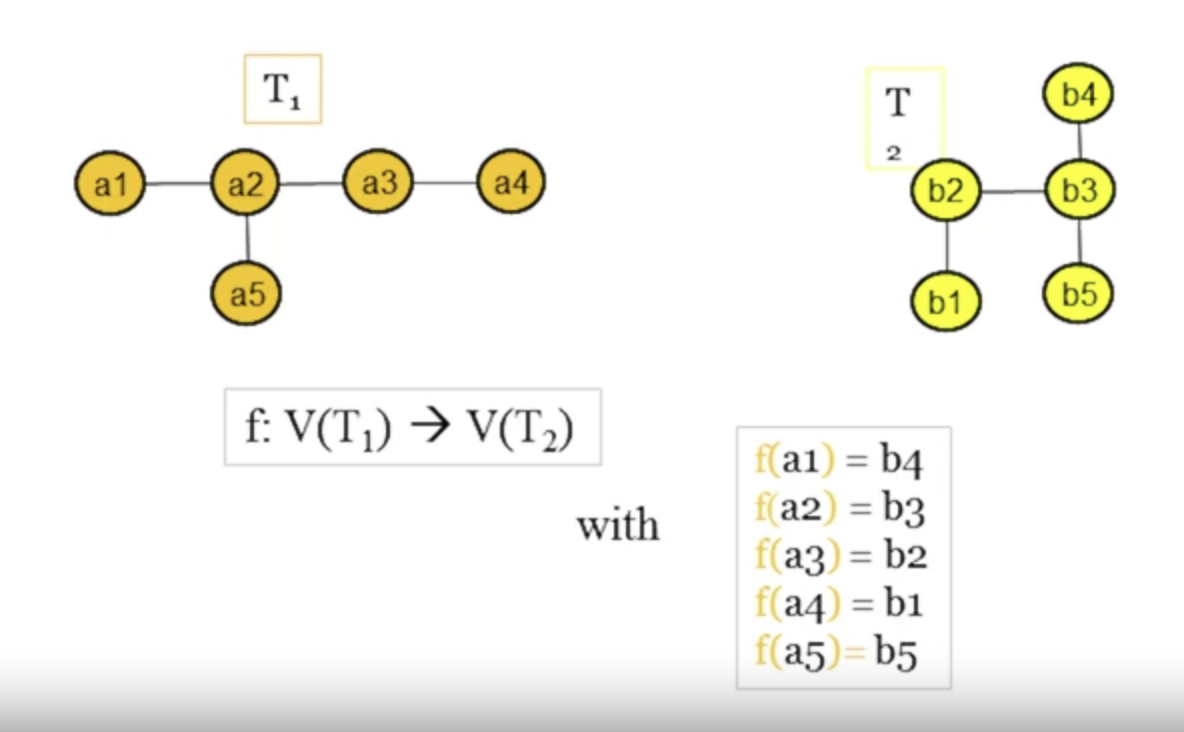• Properties

• The properties of graphs also apply to trees.
• 2 trees with different degree sequences are not isomorphic.
• 2 trees with the same degree sequence are not necessarily isomorphic.
• Isomorphic rooted trees

• Two isomorphic trees are isomorphic as rooted trees if and only if there is a bijection that maps the root of one tree to the root of the other.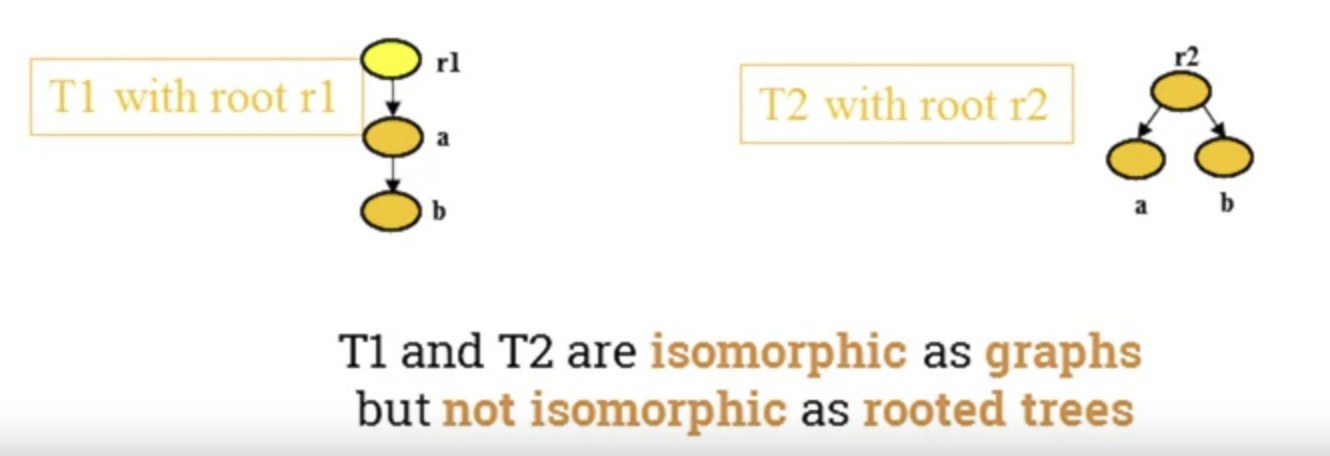## 8.203 Binary search trees

• A binary search tree with labels where they're larger on the right-hand side of the subtree and smaller on the left-hand side.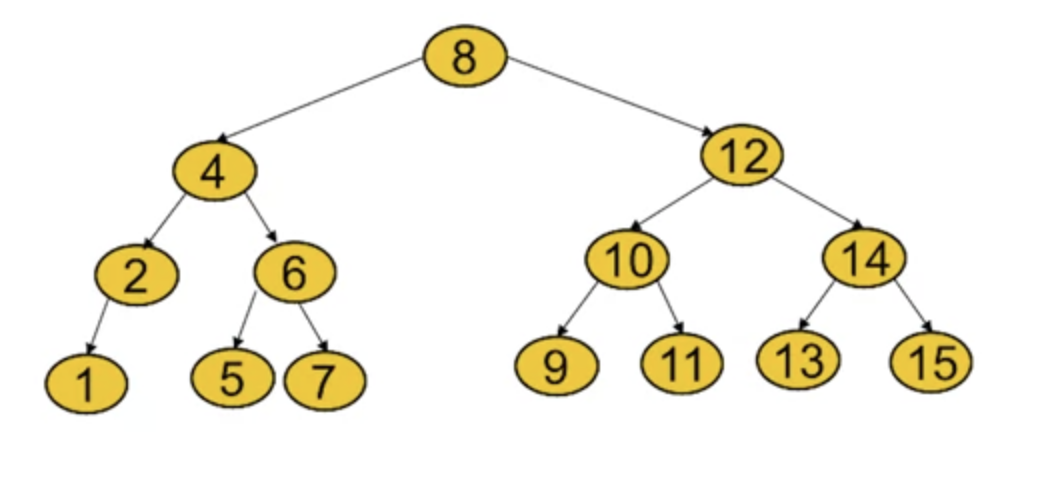• Applications
• It's useful when we want to store a modifiable collection in a memory and be able to search, insert or remove elements from the collection efficiently.
• Binary search trees can be used to solve these kinds of problems.
• Example

• Build a binary search tree to store 15 records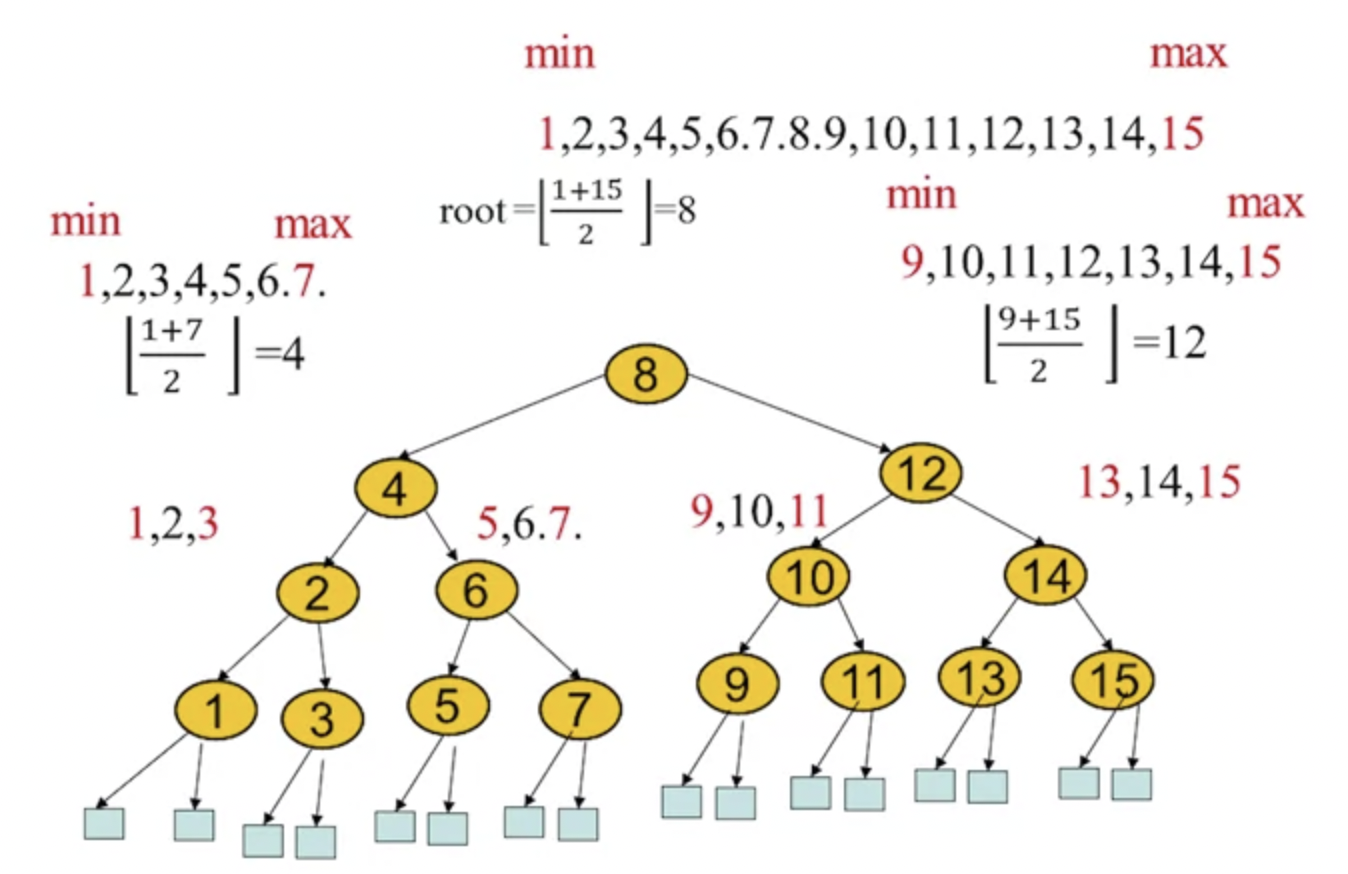• Height of a bst

• 2 methods for finding the height: * Method #1 $2^{h-1} < 1 + N \leq 2^h$ $\equiv$ $h-1 < log2(1 + N) \leq h$ * Method #2 $h = [log_2 (N + 1)]$
• Binary search algorithm
• Starts by comparing the search element to the middle term in the list.
• The list is then split into 2 smaller sub-lists of the same size, or where one of these smaller lists has one fewer term than the other.
• Search continues by restricting the search to appropriate sub-list.

## Peer Review

### Question 1

Consider the following graph, G, with 4 vertices, $x$, $y$, $z$ and $w$.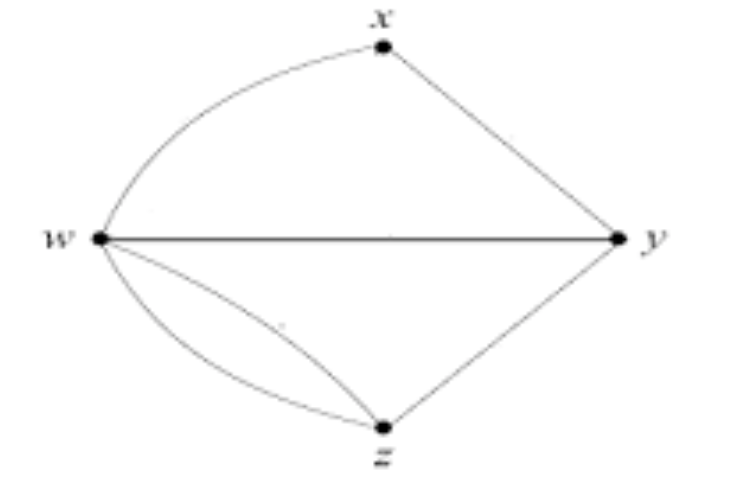1. Find the degree sequence of the graph $G$.

4, 3, 3, 2

1. Draw two non-isomorphic spanning trees of G.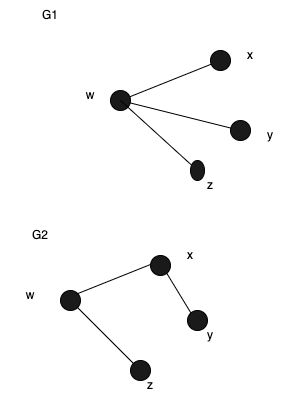1. Let $A$ be the adjacency matrix of $G$. Write down $A$.

$$A = \begin{bmatrix} \ & w & x & y & z \ w & 0 & 1 & 1 & 2 \ x & 1 & 0 & 1 & 0 \ y & 1 & 1 & 0 & 1 \ z & 2 & 0 & 1 & 0 \end{bmatrix}$$

1. What information does the sum of elements in A tell you about G?

The sum of elements is: $1 + 1 + 2 + 1 + 1 + 1 + 1 + 1 + 2 + 1 = 12$ From that information, we know that the numbers of edges is 12 / 2 = 6